## Equations for finding seconds/line in SunVox

The Handle
Posts: 185
Joined: Wed Sep 07, 2011 5:11 am

### Equations for finding seconds/line in SunVox

First off, DON'T FEEL INTIMIDATED BY THE LENGTH OF THIS POST. Most of it is algebra, changing units, and proving that the equations work. Also, half the post is pictures.These equations are useful for getting the appropriate song settings for extreme sound design. They increase your control over when pitch slides, parameter changes, etc. happen and for how long, making them perfect for helping with the creation of samples. They relate the bpm, ticks/line, and seconds/line to each other in 3 equations.

Variables:
X = Beats in a minute (ex: for 120 beats per minute, "X" would be 120 )
Y = number of ticks per line (ex: for 3 ticks/line, "Y" would be 3)
Z = seconds per line

Equations:
Z = (60 * Y) / (24 * X)
X = (60 * Y) / (24 * Z)
Y = (24 * Z * X) / 60

How I got "Z = (60 * Y) / (24 * X)":

Given:
Settings for song1: 120 bpm, 6 ticks/line, 4 lines/beat
Settings for song2: 120 bpm, 3 ticks/line, 8 lines/beat

Please note that the number of lines per beat is obtained through direct observation in SunVox.

Find:
Seconds/beat
Ticks/beat
Seconds/line

Work:
Seconds/beat for song1 and song2:
(120 beats / 1 minute) * (1 minute / 60 seconds) = 120 beats / 60 seconds = 2 beats / 1 second = 2 beats/second

We can use the reciprocal (the reverse) of the fraction representing bpm to get seconds/beat.

(1 minute / 120 beats) * (60 seconds / 1 minute) = 60 seconds / 120 beats = 1 second / 2 beats = 0.5 seconds/beat
Since the bpm is the same for song1 and song2, 0.5 seconds/beat is true for both of them.

Ticks/beat:
Song1: (6 ticks / 1 line) * (4 lines / 1 beat) = 24 ticks / 1 beat
Song2: (3 ticks / 1 line) * (8 lines / 1 beat) = 24 ticks / 1 beat

You can see that the ticks per beat remains constant. We can use the reciprocal (the reverse) of this fraction to cancel out "beats" in the following equation:

(60 seconds / 120 beats) * (1 beat / 24 ticks) = (60 seconds / [120 * 24] ticks) = 60 seconds / 2880 ticks = 0.0208333333 seconds/tick

But seconds/tick isn't what we want. We want seconds/line, so we have to do more work:

For song1:
(60 seconds / 2880 ticks) * (6 ticks / 1 line) = (60 seconds * 6) / 2880 lines = 360 seconds / 2880 lines = 0.125 seconds/line
Since it's 0.5 seconds/beat and 4 lines/beat for song1...
(0.125 seconds / 1 line) * (4 lines / 1 beat) = 0.5 seconds/beat

For song2:
(60 seconds / 2880 ticks) * (3 ticks / 1 line) = (60 seconds * 3) / 2880 lines = 180 seconds / 2880 lines = 0.0625 seconds/line
Since it's 0.5 seconds/beat and 8 lines/beat for song2...
(0.0625 seconds / 1 line) * (8 lines / 1 beat) = (0.0625 seconds * 8 ) / 1 beat = 0.5 seconds/beat

Now we can replace bpm, ticks/line, and seconds/line with variables, which gives us our equation:
Z = (60 * Y) / (24 * X)

So let's test it using song1!

Given:
Settings for song1: 120 bpm, 6 ticks/line, 4 lines/beat
(from these given settings we know that X = 128 beats and Y = 6 ticks/line)

Find:
Seconds/line

Equations:
Z = (60 * Y) / (24 * X)

Work:
Z = (60 seconds * 6 ticks/line) / (24 ticks/beat * 120 beats) = (360 seconds/line) / 2880 = 0.125 seconds/line
"Ticks" and "beats" get cancelled out, leaving "seconds/line" as the units for the result. The result we get matches up with our work from earlier.

Just in case, let's test the equation when all the variables are known. If it works, both sides should result in the same number.

0.125 seconds/line = (60 seconds * 6 ticks/line) / (24 ticks/beat * 120 beats)
0.125 seconds/line = (360 seconds/line) / 2880
0.125 seconds/line = 0.125 seconds/line

So it works! Now we need to get the other two equations. Don't feel intimidated though, because now all we have to do is some algebra to move the variables around.
I'll explain what I'm doing in parenthesis ().

Z = (60 * Y) / (24 * X)

(Multiply both sides by "X")

Z * X = (60 * Y) / 24

(Divide both sides by "Z")

X = (60 * Y) / (24 * Z)

And BAM! We've got our second equation! This one is solving for "X", the number of beats in a minute. So if we know Y (ticks/line) and Z (seconds/line), we can solve for "X".

Now let's get the last equation:

X = (60 * Y) / (24 * Z)

(Multiply both sides by "(24 * Z)")

(24 * Z * X) = (60 * Y)

(Divide both sides by 60)

(24 * Z * X) / 60 = Y
Y = (24 * Z * X) / 60

So now we have our last equation! This one is solving for "Y", the number of ticks per line. So if we know X (beats in a minute) and Z (seconds/line), we can solve for "Y".

I'm assuming you're thinking something like "But Handle, wtf am I going to use these for?" Here's an example:

Suppose I want to recreate (or make something similar to) a sound from my favorite track, and I want to do this with SunVox. I want to get really close to the desired sound (and I can measure time in milliseconds with audacity), so I'll need to be as accurate as possible in the timing of pitch slides, effects, etc.

In order to get that timing to be accurate, I have to know how long each line in SunVox lasts....but since there's nothing indicating the time spent per line, I'd either have to guess or make equations (as I have done above).

So let's suppose I want each line to last 0.005 seconds...

Given:
Z = 0.005 seconds/line

Find:
BPM
ticks/line

Equations:
Z = (60 * Y) / (24 * X)

Work:
0.005 seconds/line = (60 * Y) / (24 * X)

(Multiply both sides by "(24 / 60)")

0.002 seconds/line = Y / X

(0.002 = 1/500)

(1/500) seconds/line = Y / X

So now we know that Y = 1, and X = 500. So we can set the ticks/line to 1, and the bpm to 500. This will get us the 0.005 seconds/line that we wanted.

But don't take my word for it, try it yourself!

This example will show that when you export the track (4 lines long) when the bpm is 500 and ticks/line is 1, the length of the exported track will be 0.020 seconds (0.005 seconds/line).

1.) Set BPM to 500 and ticks/line to 1.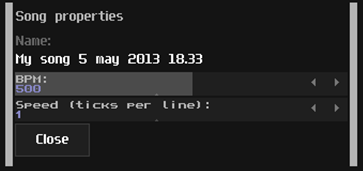2.) Change the length of the pattern to 4 lines.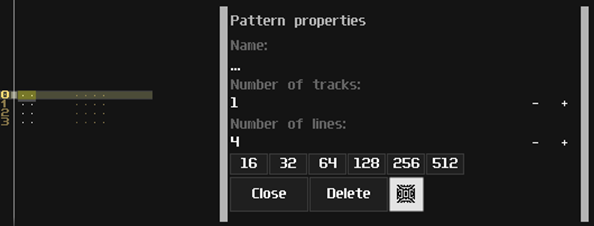3.) Export the track to WAV. Your export settings shouldn't matter as we are only interested in the length of the exported track.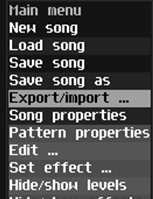4.) Import the track to Audacity.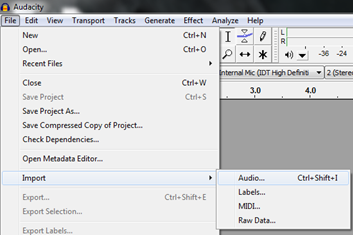5.) Right click the middle dropdown at the bottom to make sure the units are set to show milliseconds.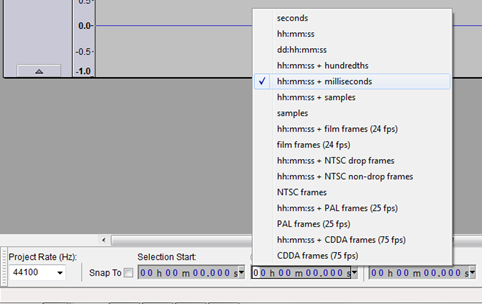6.) Double click on the imported track to show the exact length of it (length shown at bottom where you changed the units).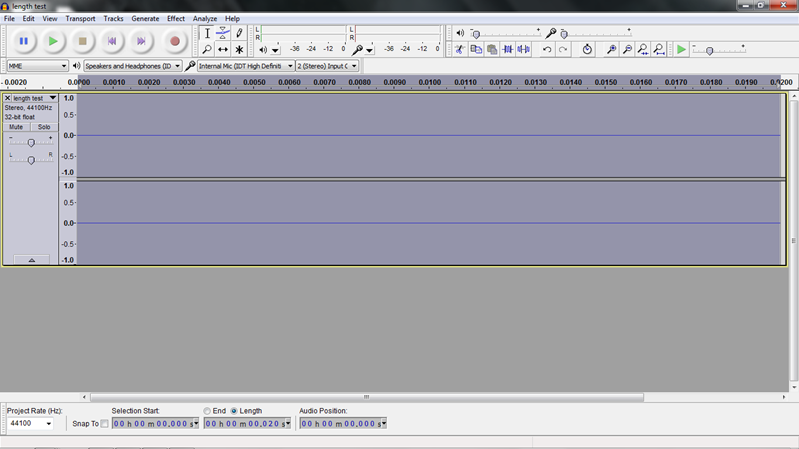odoyle
Posts: 30
Joined: Fri May 10, 2013 9:17 pm

### Re: Equations for finding seconds/line in SunVox

Oh, my word dude. I need a Ritalin just to get five minutes into this post, lol